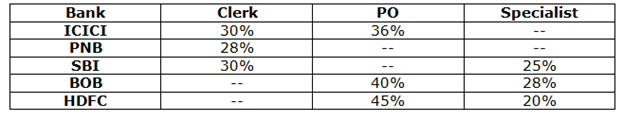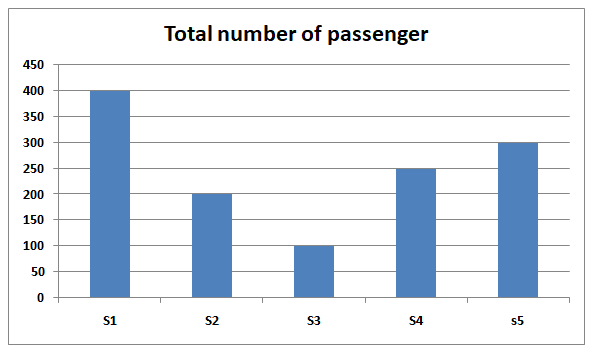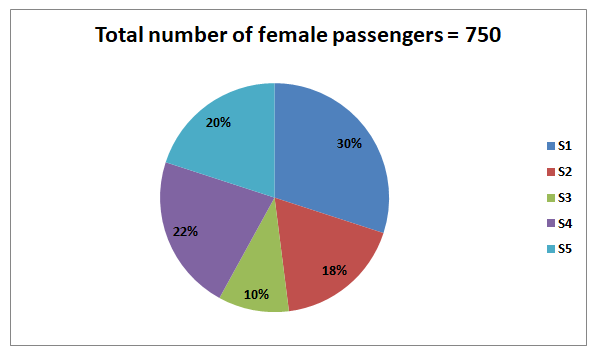# RRB PO Mains Quantitative Aptitude 2021 (Day-02)

Dear Aspirants, Our IBPS Guide team is providing new series of Quantitative Aptitude Questions for RRB PO 2021 Mains so the aspirants can practice it on a daily basis. These questions are framed by our skilled experts after understanding your needs thoroughly. Aspirants can practice these new series questions daily to familiarize with the exact exam pattern and make your preparation effective.

### Start Quiz

Missing DI

Directions (01-05): Study the following information carefully and answer the questions given below.

The given table graph shows the percentage of employee (PO, Clerk and Specialist) out of total employees in five different banks.1) If the ratio of the total number of employees in ICICI and PNB is 10:7 and the total number of employees in ICICI is 3600 more than the number of employees in PNB, then what is the difference between the number of Clerk in ICICI and PNB?

A.1248

B.1252

C.1244

D.1240

E.1336

2) Ratio of the number of PO in PNB and SBI is 28:15 and the ratio of the number of specialist to clerk in PNB is 15:14. If the total number of employees in PNB and SBI together is 7200, then what is the difference between the number of specialist in SBI and PNB?

A.820

B.840

C.780

D.740

E.880

3) The average salary of the specialist in ICICI is Rs.80 and the ratio of the total number of employees in ICICI and BOB is 6:7. If the total salary of all the specialist together in ICICI is Rs.97920, then what is the difference between the number of PO in ICICI and BOB?

A.384

B.386

C.388

D.392

E.394

4) If the total number of clerk in HDFC and SBI is 3630 and the total number of employees in HDFC is 50% more than the total number of employees in SBI, then what is the sum of the number of specialist in SBI and HDFC?

A.2440

B.2450

C.2420

D.2400

E.2460

5) The number of employees in HDFC is 80% more than the number of employees in BOB and the ratio of the number of male to female PO in HDFC and BOB is 5:4 and 5:3 respectively. The number of male PO employees in BOB is what percent of the number of female PO employees in HDFC?

A.78.44%

B.71.44%

C.67.44%

D.69.44%

E.Cannot be determined

Mixed DI

Directions (06-10): Study the following information carefully and answer the questions given below.

The given bar graph shows the number of passengers in 5 coaches of a train.The given pie chart shows the percentage distribution of the female passengers in 5 coaches of a train.6) What is the ratio of the number of male passengers in S1 and S2 coaches together to the number of female passengers in S3 and S4 together?

A.2:1

B.3:2

C.1:2

D.1:1

E.2:3

7) The number of male passengers in S3 and S4 together is what percent of the total number of passengers in S3 and S4 together?

A.31.43%

B.43.84%

C.27.87%

D.35.28%

E.45.21%

8) What is the difference between the total number of male passengers in S2, S3 and S4 together and the total number of female passengers in S1 and S5 together?

A.180

B.200

C.240

D.150

E.225

9) The number of female passengers in S2 and S5 together is what percent more than the number of female passengers in S3 and S4?

A.12.5%

B.15.75%

C.10.50%

D.18.75%

E.20.25%

10) If the number of male passengers in S6 is 40% more than the number of male passengers in S5 and the number of female passengers in S6 is 50% more than the number of male passengers in the same coach, then what is the total number of passengers in S6?

A.485

B.510

C.495

D.515

E.525

Number of employees in ICICI = 10/3 * 3600 = 12000

Number of employees in PNB = 7/3 * 3600 = 8400

Number of clerk in ICICI = 12000 * 30/100 = 3600

Number of clerk in PNB = 8400 * 28/100 = 2352

Difference = 3600 – 2352 = 1248

Number of employees in PNB = 100x

Number of specialist in PNB = 15/14 * 28 = 30%

Number of PO in PNB = 100x * (100 – 28 – 30)/100 = 42x

Number of employees in SBI = 100y

Number of PO in SBI = 100y * (100 – 30 – 25)/100 = 45y

42x/45y = 28/15

x/y = 2/1

100x + 100(x/2) = 7200

x = 48

Number of employees in SBI = 48/2 * 100 = 2400

Number of employees in PNB = 48 * 100 = 4800

Specialist in PNB = 4800 * 30/100 = 1440

Specialist in SBI = 2400 * 25/100 = 600

Difference = 1440 – 600 = 840

Number of employees in ICICI = 6x

Number of employees in BOB = 7x

6x * 34/100 * 80 = 97920

x = 600

Number of PO in ICICI = 600 * 6 * 36/100 = 1296

Number of PO in BOB = 600 * 7 * 40/100 = 1680

Difference = 1680 – 1296 = 384

Number of employees in SBI = 2x

Number of employees in HDFC = 2x * 150/100 = 3x

Number of clerk in HDFC = 3x * (100 – 45 – 20)/100 = 1.05x

Number of clerk in SBI = 2x * 30/100 = 0.6x

0.6x + 1.05x = 3630

x = 2200

Number of specialist in SBI = 2200 * 2 * 25/100 = 1100

Number of specialist in HDFC = 2200 * 3 * 20/100 = 1320

Required sum = 1100 + 1320 = 2420

Number of employees in BOB = 100x

Total number of employees in HDFC = 100x * 180/100 = 180x

Number of male PO employees in BOB = 5/8 * 40/100 * 100x = 25x

Number of female PO employees in HDFC = 180x * 45/100 * 4/9 = 36x

Required percentage = 25x/36x * 100

= 69.44%

Total number of passenger in S1 = 400

Number of female passengers in S1 = 30 * 750/100 = 225

Number of male passengers in S1 = 400 – 225 = 175

Total number of passengers in S2 = 200

Number of female passengers in S2 = 18/100 * 750 = 135

Number of male passengers in S2 = 200 – 135 = 65

Total number of passengers in S3 = 100

Number of female passengers in S3 = 10/100 * 750 = 75

Number of male passengers in S3 = 100 – 75 = 25

Total number of passengers in S4 = 250

Number of female passengers in S4 = 22/100 * 750 = 165

Number of male passengers in S4 = 250 – 165 = 85

Total number of passengers in S5 = 300

Number of female passengers in S5 = 20/100 * 750 = 150

Number of male passengers in S5 = 300 – 150 = 150

Required ratio = (175 + 65):(75 + 165)

= 240:240

= 1:1

Required percentage = (25 + 85)/(100 + 250) * 100

= (110/350) * 100

= 31.43%

Required difference = (225 + 150) – (65 + 25 + 85)

= 375 – 175

= 200

Required percentage = (285-240)/240* 100

= (45/240) * 100

= 18.75%